# ข้อสอบ CU-ATS Chemistry พร้อมเฉลย มาทดสอบกันพร้อมสอบแค่ไหน?

## CU-ATS Chemistry

1. From the list of standard electrode potentials shown below, what is the strongest oxidizing and reducing reagents?

VO2++ 2H+ + e- V3+ + H2O             Eo = + 0.34 V

S2O32- + 6H+ + 4e- ⇌ 2S + 3H2O        Eo = + 0.47 V

ClO- + H2O + 2e- Cl- + 2OH-            Eo = + 0.89 V

VO2+ + 2H+ + e- VO2+ + H2O          Eo = + 1.00 V

 Strongest oxidizing agent Strongest reducing agent 1) VO2+ S2O32- 2) S2O32- VO2+ 3) VO2+ ClO- 4) ClO- V3+ 5) VO2+ V3+
2. The graph above shows a relationship between vapor pressure and the temperature of ether, water, and mercury. Which substance has the lowest surface tension?

3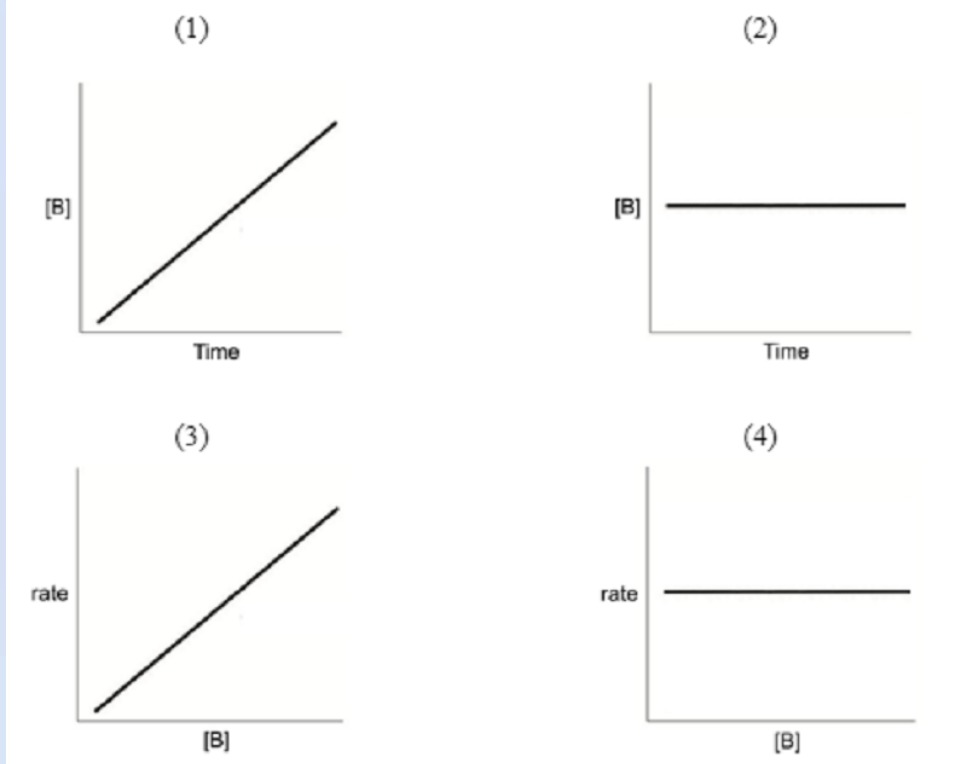4. A balloon has a volume of 20.0 dm3 at the mean sea level (1 atm and 27 °C). It rises to the top of the mountain, which is 1 km higher from the mean sea level, and explodes. At the top of the mountain, the external pressure is 0.5 atm and the temperature reduces to -23 °C. What is the largest volume of the balloon at the top of the mountain?
5. What is the pH value of basic salt of 1.0 M Na2X, when Ka1 and Ka2 of H2X = 10-8 and 10-10, respectively?
6. Which of the following substances has the highest boiling point? (Relative atomic mass of H = 1, C = 12, O = 16)
7. A reversible reaction A + B 2C has the equilibrium constant, K, equals to 9. A and B have the same initial concentrations of 0.02 M. What is the concentration of B at equilibrium?
8. Dimethandrolone undecanoate (DMAU) is a compound being developed for use as a potential male hormonal contraceptive, specifically as a birth control pill for men. From the molecular structure of DMAU shown below, which geometry can be found the most in carbon atoms of this molecule?
9. A student carried out an experiment by adding an unknown substance B to a test-tube containing Benedict’s solution. When the test-tube was heated, the solution remained blue. In a separate test-tube containing dilute hydrochloric acid, substance B was added to the test-tube, which was then heated in boiling water for 10 minutes. The solution was neutralised before being added to a test-tube containing Benedict’ solution, which was then heated. Brick red precipitates were observed. What could the unknown B be?
10. Element X has a relative atomic mass 14 times greater than that of tritium and the number of neutrons 22 times greater than that of deuterium. What is the property of the oxide of X?
11. What is the electronic configuration of cobalt in the compound [Co(NH3)4SO4]NO3?
(Proton number of Co = 27)
12. What is the density, in the unit of kg m-3, of this metal with the mass of 31.5 g?
13. The table below shows some radioisotopes with their half-life.

 Radioisotope Half-life (days) Cobalt-57 271.79 Einsteinium-254 275.7 Berkelium-249 330 Californium-248 333.5

Mass of oisotope Z decreases from 5.44 g to 0.34 g after 3.655 years.

Given that a year has 365 days. What is the identity of the radioisotope Z?

14. Which five gases are compressed into each balloon which contains 2 liters. Which one of the following gases makes the balloons still the largest size when hanging each balloon at room temperature after passing two weeks? (Relative atomic mass of H = 1, He = 4, Ne = 10, N = 14, O = 16, Ne = 20)
15. Which of the following statements about absolute zero is NOT TRUE?
16. What is the major difference between DNA and RNA?
17. Which of the following is the molecular formula C7H10?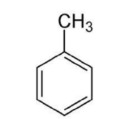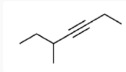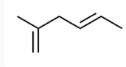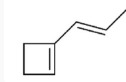18. Which of the following pairs of substances can form a buffer solution when being mixed?
19. What is the chemical formula of limestone?
20. Consider the following reaction;

2A + (5/2)B2 → A2B5

If the rate of formation of A2B5 is r M/s, what is the rate at which B2 is consumed?

CU-ATS Chemistry
You got {{userScore}} out of {{maxScore}} correct
{{title}}
{{image}}
{{content}}